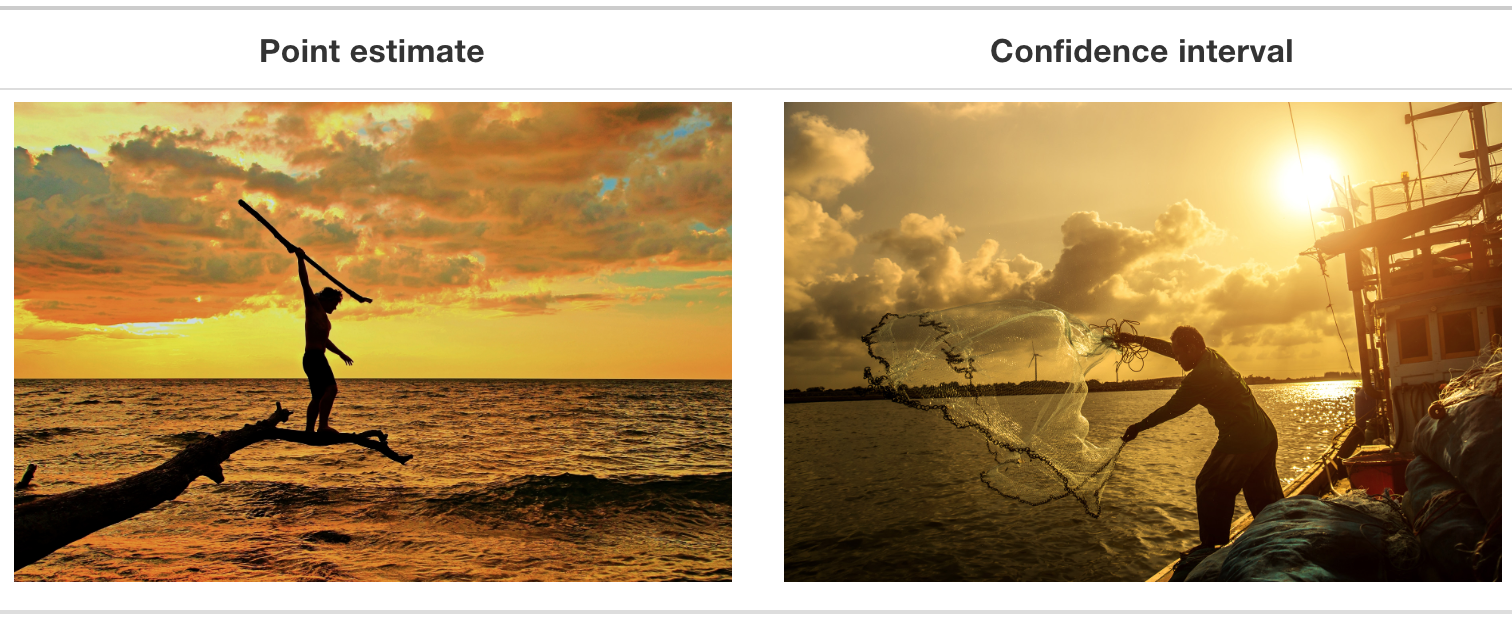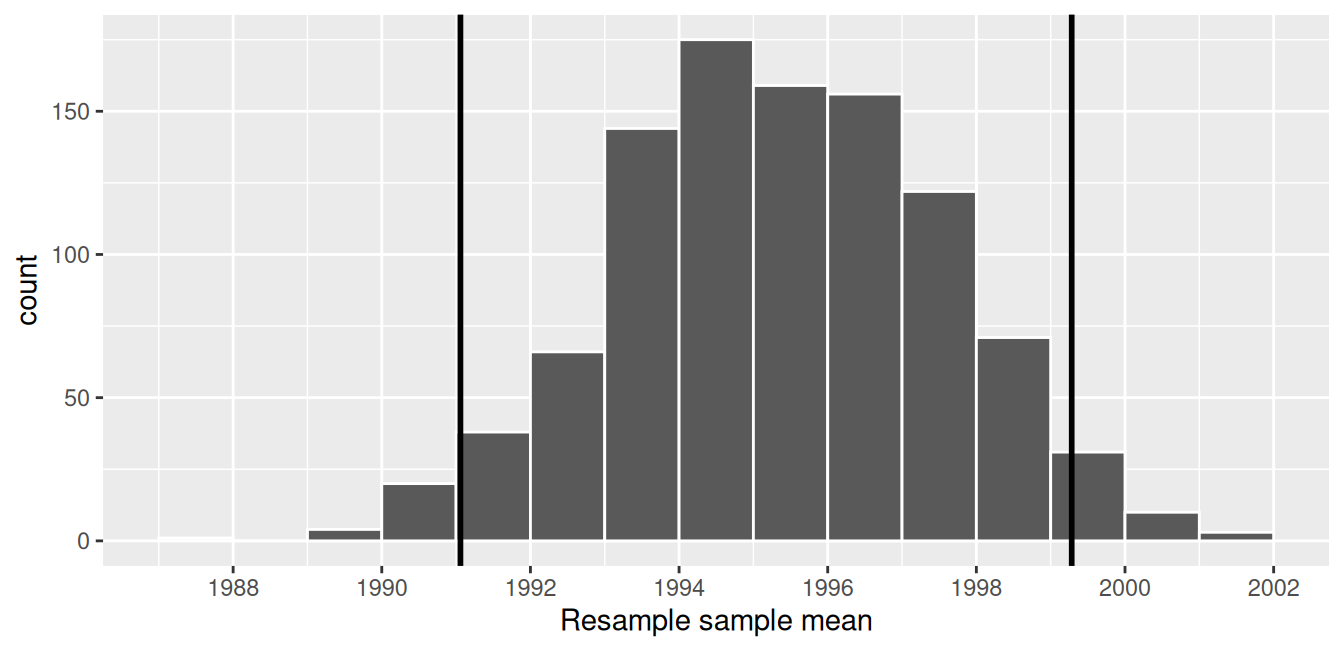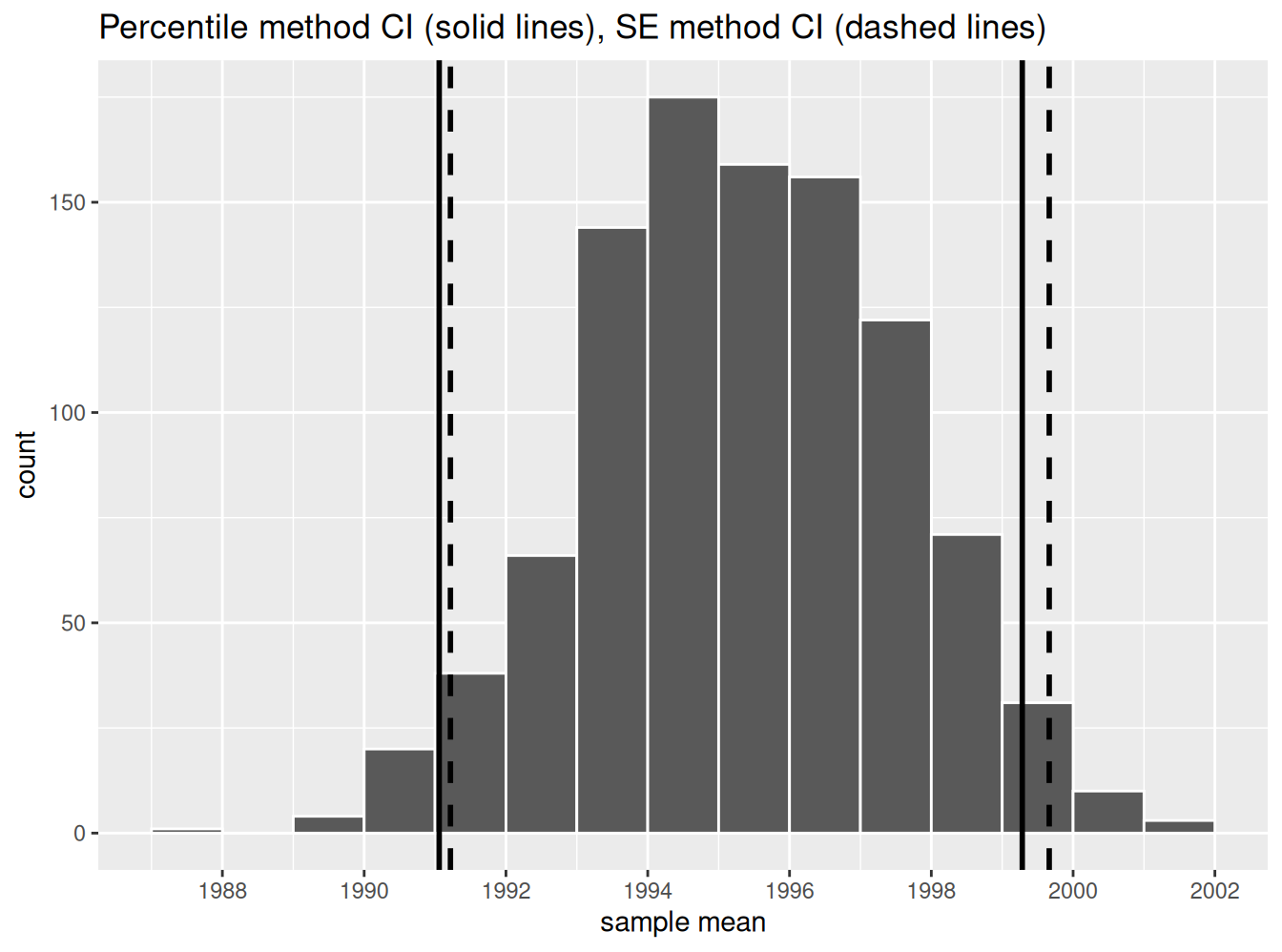## 8.3 Understanding confidence intervals

Let’s start this section with an analogy involving fishing. Say you are trying to catch a fish. On the one hand, you could use a spear, while on the other you could use a net. Using the net will probably allow you to catch more fish!

Now think back to our pennies exercise where you are trying to estimate the true population mean year $$\mu$$ of all US pennies. Think of the value of $$\mu$$ as a fish.

On the one hand, we could use the appropriate point estimate/sample statistic to estimate $$\mu$$, which we saw in Table 8.1 is the sample mean $$\overline{x}$$. Based on our sample of 50 pennies from the bank, the sample mean was 1995.44. Think of using this value as “fishing with a spear.”

What would “fishing with a net” correspond to? Look at the bootstrap distribution in Figure 8.14 once more. Between which two years would you say that “most” sample means lie? While this question is somewhat subjective, saying that most sample means lie between 1992 and 2000 would not be unreasonable. Think of this interval as the “net.”

What we’ve just illustrated is the concept of a confidence interval, which we’ll abbreviate with “CI” throughout this book. As opposed to a point estimate/sample statistic that estimates the value of an unknown population parameter with a single value, a confidence interval gives what can be interpreted as a range of plausible values. Going back to our analogy, point estimates/sample statistics can be thought of as spears, whereas confidence intervals can be thought of as nets.FIGURE 8.15: Analogy of difference between point estimates and confidence intervals.

Our proposed interval of 1992 to 2000 was constructed by eye and was thus somewhat subjective. We now introduce two methods for constructing such intervals in a more exact fashion: the percentile method and the standard error method.

Both methods for confidence interval construction share some commonalities. First, they are both constructed from a bootstrap distribution, as you constructed in Subsection 8.2.3 and visualized in Figure 8.14.

Second, they both require you to specify the confidence level. Commonly used confidence levels include 90%, 95%, and 99%. All other things being equal, higher confidence levels correspond to wider confidence intervals, and lower confidence levels correspond to narrower confidence intervals. In this book, we’ll be mostly using 95% and hence constructing “95% confidence intervals for $$\mu$$” for our pennies activity.

### 8.3.1 Percentile method

One method to construct a confidence interval is to use the middle 95% of values of the bootstrap distribution. We can do this by computing the 2.5th and 97.5th percentiles, which are 1991.059 and 1999.283, respectively. This is known as the percentile method for constructing confidence intervals.

For now, let’s focus only on the concepts behind a percentile method constructed confidence interval; we’ll show you the code that computes these values in the next section.

Let’s mark these percentiles on the bootstrap distribution with vertical lines in Figure 8.16. About 95% of the mean_year variable values in virtual_resampled_means fall between 1991.059 and 1999.283, with 2.5% to the left of the leftmost line and 2.5% to the right of the rightmost line.FIGURE 8.16: Percentile method 95% confidence interval. Interval endpoints marked by vertical lines.

### 8.3.2 Standard error method

Recall in Appendix A.2, we saw that if a numerical variable follows a normal distribution, or, in other words, the histogram of this variable is bell-shaped, then roughly 95% of values fall between $$\pm$$ 1.96 standard deviations of the mean. Given that our bootstrap distribution based on 1000 resamples with replacement in Figure 8.14 is normally shaped, let’s use this fact about normal distributions to construct a confidence interval in a different way.

First, recall the bootstrap distribution has a mean equal to 1995.36. This value almost coincides exactly with the value of the sample mean $$\overline{x}$$ of our original 50 pennies of 1995.44. Second, let’s compute the standard deviation of the bootstrap distribution using the values of mean_year in the virtual_resampled_means data frame:

virtual_resampled_means %>%
summarize(SE = sd(mean_year))
# A tibble: 1 x 1
SE
<dbl>
1 2.15466

What is this value? Recall that the bootstrap distribution is an approximation to the sampling distribution. Recall also that the standard deviation of a sampling distribution has a special name: the standard error. Putting these two facts together, we can say that 2.155 is an approximation of the standard error of $$\overline{x}$$.

Thus, using our 95% rule of thumb about normal distributions from Appendix A.2, we can use the following formula to determine the lower and upper endpoints of a 95% confidence interval for $$\mu$$:

\begin{aligned} \overline{x} \pm 1.96 \cdot SE &= (\overline{x} - 1.96 \cdot SE, \overline{x} + 1.96 \cdot SE)\\ &= (1995.44 - 1.96 \cdot 2.15, 1995.44 + 1.96 \cdot 2.15)\\ &= (1991.15, 1999.73) \end{aligned}

Let’s now add the SE method confidence interval with dashed lines in Figure 8.17.FIGURE 8.17: Comparing two 95% confidence interval methods.

We see that both methods produce nearly identical 95% confidence intervals for $$\mu$$ with the percentile method yielding $$(1991.06, 1999.28)$$ while the standard error method produces $$(1991.22, 1999.66)$$. However, recall that we can only use the standard error rule when the bootstrap distribution is roughly normally shaped.

Now that we’ve introduced the concept of confidence intervals and laid out the intuition behind two methods for constructing them, let’s explore the code that allows us to construct them.

Learning check

(LC8.3) What condition about the bootstrap distribution must be met for us to be able to construct confidence intervals using the standard error method?

(LC8.4) Say we wanted to construct a 68% confidence interval instead of a 95% confidence interval for $$\mu$$. Describe what changes are needed to make this happen. Hint: we suggest you look at Appendix A.2 on the normal distribution.